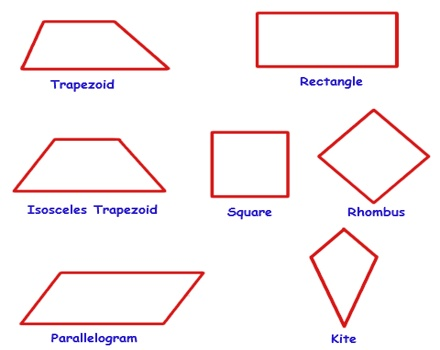A quadrilateral is a closed figure with four straight sides. Each point where two sides touch is called a vertex. Simple quadrilaterals are either convex or concave.

Properties

• Four sides (edges)
• Four vertices (corners)
• The interior angles add up to 360 degreesTypes of Quadrilaterals

There are special types of quadrilateral:

Rectangle: A rectangle is a four-sided shape where every angle is a right angle (90°). It is a quadrilateral with four right angles; a rectangle is a type of parallelogram

Rhombus: A rhombus is a four-sided shape where all sides have equal length. It is quadrilateral with four congruent sides; a rhombus is both a kite and a parallelogram.

Square: A square has equal sides and every angle is a right angle (90°). it is a quadrilateral with four congruent sides and four right angles; a square is both a rhombus and a rectangle

Parallelogram: A parallelogram has opposite sides parallel and equal in length. Also opposite angles are equal. It is quadrilateral that has two pairs of parallel sides

Trapezoid / Trapezium: A trapezoid / trapezium has a pair of opposite sides parallel. It is a quadrilateral with exactly one pair of parallel sides (the parallel sides are called bases).

Kite: It has two pairs of sides. Each pair is made up of adjacent sides (they meet) that are equal in length.

Irregular Quadrilaterals: The only regular (all sides equal and all angles equal) quadrilateral is a square. So all other quadrilaterals are irregular.

A quadrilateral, in general, has sides of different lengths and angles of different measures. However, squares, rectangles, etc. are special types of quadrilaterals with some of their sides and angles being equal.